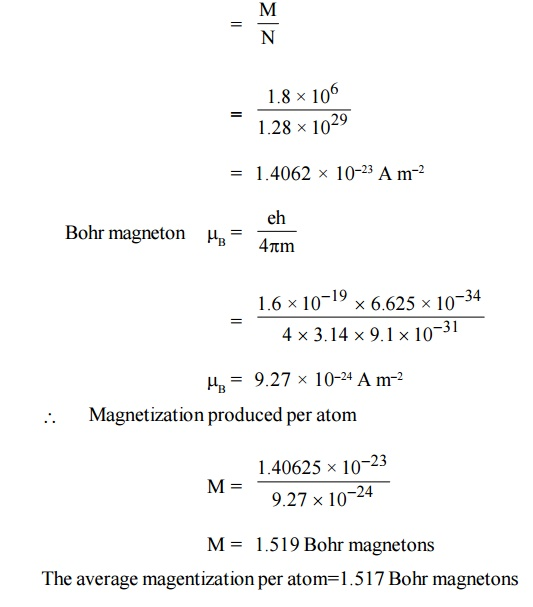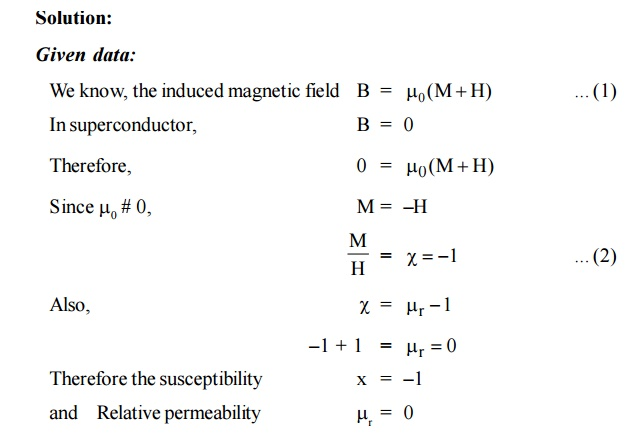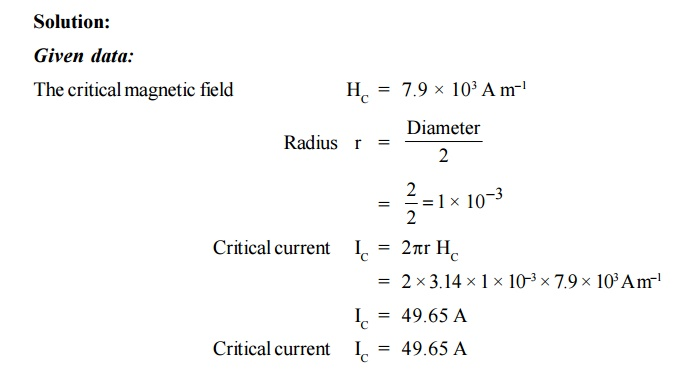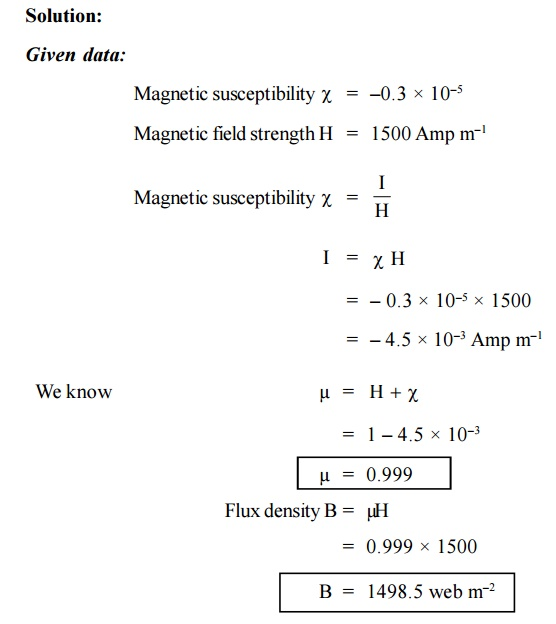Home | | Engineering Physics II | Solved Problems: Magnetic and Superconducting Materials

# Solved Problems: Magnetic and Superconducting Materials

Physics - Magnetic and Superconducting Materials - Important Solved Problems(Sum): Magnetic and Superconducting Materials

SOLVED PROBLEMS

A paramagnetic material has a magnetic field intensity of 104 Am–1. If the susceptibility of the material at room temperature is 3.7 × 10–5. Calculate the magnetization and flux density in the material.A magnetic material has a magnetization of 2300 A m–1 and produces a flux density of 0.00314 Wb m–2. Calculate the magnetizing force and the relative permeability of the material.

Solution :

Given data:

Magnetization M = 2300 A m–1

Flux density B = 0.00314 Web m–2.A paramagnetic material has FCC structure with a cubic edge of 2.5 A°. If the saturation value of magnetization is 1.8 × 106 A m–1, Calculate the magnetization contributed per atom in Bohr magnetrons.The saturation magnetic induction of Nickel is 0.65 Wb m–2. If the density of Nickel is 8906 kg m–3 and its atomic weight is 58.7, calculate the magnetic moment of the Nickel atom in Bohr magnetron.

Solution:

Given data:

Saturation magnetic induction of NickelIn a magnetic material the field strength is found to be 106 A m–1. If the magnetic susceptibility of the material is 0.5 × 10–5, calculate the intensity of magnetization and flux density in the material.A superconducting tin has a critical temperature of 3.7 K at zero magnetic field and a critical field of 0.0306 Tesla at 0 K. Find the critical field at 2 K.7.        Calculate the critical current and current density for a wire of a lead having a diameter of 1 mm at 4.2 K. The critical temperature for lead is 7.18 K and H = 6.5 × 104 A m–1.Prove that susceptibility of superconductor is -1 and relative permeability is zero.Find the critical current which can pass through a long thin superconducting wire of aluminum of diameter 2 mm, the critical magnetic field for aluminum is 7.9 × 103 A m–1.The superconducting transistion temperature of Lead is 7.26 K. The initial field at 0 K is 64 × 103 Amp m–1. Calculate the critical field at 5 K.A magnetic field of 2000 Amp m–1 is applied to a material which has a susceptibility of 1000. Calculate the (i) Intensity and (ii) Flux density.The superconducting transition temperature of lead of 7.26 K. The initial field at 0 K is 64 × 103 Amp m–1. Calculate the critical field at 5 K.The magnetic field strength of Silicon is 1500 Amp m–1. If the magnetic susceptibility is (–0.3 × 10–5), calculate the magnetization and flux density in Silicon.14. Calculate the critical current which can flow through a long thin super conducting wire of diameter 1 mm. The critical magnetic field is 7.9 × 103 Amp m–1.ASSIGNMENT PROBLEMS

1.The saturation value of magnetization of iron is 1.76 × 106 A m–1. Iron had body centered cubic structure with an elementary edge of 2.86 Å.Calculate the average number of Bohr magnetrons contributed to the magnetization per atom.

(Ans: 2.2 Bohr magnetron per atom)

2.The magnetic field intensity of a ferric oxide piece is 106 A m–1. If the susceptibility of the material at room temperature is 10.5 × 10–3, calculate the flux density and magnetization of the material.

(Ans: B = 1.259 T and M = 1500 A m–1 )

3.  A magnetic material has a magnetization of 3000 A m–1 and flux density of 0.044 Wb m–2. Calculate the magnetic force and the relative permeability of the

material.                                             (Ans: M = 203 and r = 17.26)

Calculate the magnetic filed in the lead at 5 K, if it’s critical magnetic field at 0 K H0 = 8 × 105 A m–1, and transition temperature TC = 7.26 K

(Ans: 4.2 × 105 A m–1)

The critical temperature TC for mercury with isotopic mass 199.5 is 4.185 K. Calculate its critical Temperature, when it’s isotopic masses changes to 203.4.

(Ans: 4.139 K)

Calculate the critical current which can flow though a long thin superconducting wire of aluminum of diameter 1 mm. The critical magnetic field for aluminum is 7.9 × 103 A m–1.

Study Material, Lecturing Notes, Assignment, Reference, Wiki description explanation, brief detail
Physics : Magnetic and Superconducting Materials : Solved Problems: Magnetic and Superconducting Materials |